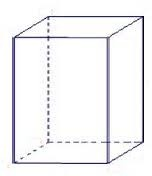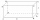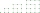# Regular prism

The regular four-sided prism has a base of 25 cm2 and a surface of 210 cm2. Find its volume.

V =  200 cm3

### Step-by-step explanation:Did you find an error or inaccuracy? Feel free to write us. Thank you!Tips to related online calculators
Tip: Our volume units converter will help you with the conversion of volume units.

#### You need to know the following knowledge to solve this word math problem:

We encourage you to watch this tutorial video on this math problem:

## Related math problems and questions:The surface of the regular quadrilateral prism is 8800 cm2, the base edge is 20 cm long. Calculate the volume of the prism
• Four prismsQuestion No. 1: The prism has the dimensions a = 2.5 cm, b = 100 mm, c = 12 cm. What is its volume? a) 3000 cm2 b) 300 cm2 c) 3000 cm3 d) 300 cm3 Question No.2: The prism base is a rhombus with a side length of 30 cm and a height of 27 cm. The height of t
• Edge of prismThe regular quadrilateral prism has a surface of 250 dm2, its shell has a content of 200 dm2. Calculate its leading edge.
• Prism 4 sidesFind the surface area and volume four-sided prism high 10cm if its base is a rectangle measuring 8 cm and 1.2dm
• PrismCalculate the surface area and volume of a prism with a body height h = 10 cm, and its base has the shape of a rhomboid with sides a = 5.8 cm, b = 3 cm, and the distance of its two longer sides is w = 2.4 cm.
• Base sideIn a quadrilateral prism, are known surface area S = 12400 mm2 base side m = 40mm and prism height = 120mm, what is the length of base side n =?Calculate the volume and surface area of a regular quadrangular prism 35 cm high and the base diagonal of 22 cm.The height of a regular quadrilateral prism is v = 10 cm, the deviation of the body diagonal from the base is 60°. Determine the length of the base edges, the surface, and the volume of the prism.
• The regularThe regular quadrilateral pyramid has a volume of 24 dm3 and a height of 45 cm. Calculate its surface.What is the volume of a regular quadrilateral pyramid if its surface is 576 cm2 and the base edge is 16 cm?The quadrangular prism has a volume of 648 cm3. Trapezoid which is its base has the dimensions bases: a = 10 cm, c = 5 and height v = 6 cm. What is the height of the prism?Calculate surface and volume tetrahedral prism, which has a rhomboid-shaped base, and its dimensions are: a = 12 cm, b = 7 cm, ha = 6 cm and prism height h = 10 cm.Find the surface area of a regular quadrilateral pyramid which has a volume of 24 dm3 and a height of 45 cm.The perimeter of a quadrilateral (four sided plane shape) is 125 cm . If the three known sides measure a=12 cm, b=45 cm, and c=30.5 cm, how long is the fourth side?A quadrilateral pyramid, which has a rectangular base with dimensions of 24 cm, 13 cm. The height of the pyramid is 18cm. Calculate 1/the area of the base 2/casing area 3/pyramid surface 4/volume of the pyramidThe regular hexagonal prism has a surface of 140 cm2 and height of 5 cm. Calculate its volume.The cube has an area of base 256 mm2. Calculate the edge length, volume, and area of its surface.